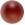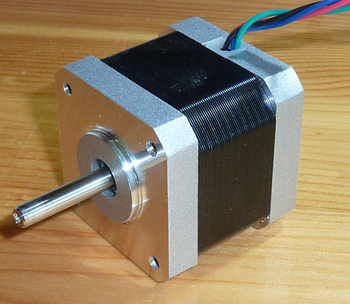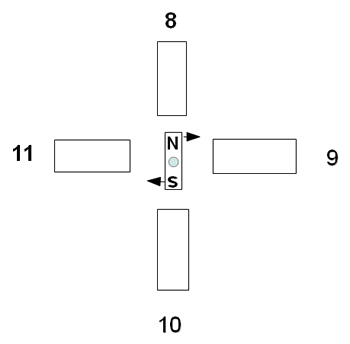Back

Stepper motors

All electric motors produce forces by the repulsion and attraction of two magnetic fields. In rotary motors one field is on the rotating part, called the rotor, and the other is on the body of the motor, the stator. Sometimes both fields are made by a current flowing though coils. Sometimes the rotor or the stator is a permanent magnet, these days often a highly magnetic one made of neodymium alloy.

An ordinary rotary motor turns continuously when electric currents are put into it. The speed and/or power increases as the current does. Speed is measured in revolutions per minute (rpm). Power is measured in watts or horsepower. One horsepower is about 750 watts. Another important measure for a motor is its turning force, called torque. This is measured as a force (kg, gram, newton etc) multiplied by a distance (m, cm etc). For example a motor might have a torque of 10 cm N (centimetre newton). This would mean that it can produce a force of 10 N using a pulley with a radius of 1 cm or a force of 0.1 N at the end of a 100 cm lever. Note that in the USA the old imperial units of pound or ounce forces and inch or foot distances are still used in engineering.

A stepper motor is different. There are two pairs of coils called phases. When one pair has a current in it (energised) a magnetised rotor turns to line up with it. If the other pair is now energised the rotor steps round to it. If both pairs are energised the rotor steps to half-way between them. By energising one pair, then two, then the second one, then both, then the first one and so on, the rotor steps round. So a continuous sequence of these energisations causes continuous rotation. The benefits of a stepper are that it stops when you stop the sequence and it can be positioned accurately by giving a known number of steps. If you maintain the current when stopped the motor strongly resists moving out of its position. If not it has a lower resistance called the detent force.

In practice the rotor is cog-shaped so it gives many steps for each revolution. A typical value for a step is 1.8 degrees angle, giving 200 in one revolution. Combined with gearing this can give the very fine movements needed for example for machine tool cutter or drill positioning or printing three dimensional objects. There are excellent animations on http://pcbheaven.com/wikipages/How_Stepper_Motors_Work/ .

Here is a typical smallish stepper. In this case it conforms to the NEMA (US National Electrical Manufacturers Association) specifications. See http://www.nema.org

The specifications also include voltages and coil resistances. These are irrelevant, as the controller board sets the current to be whatever is required, so I have not included them.

This what it looks like.Frame Size NEMA17 NEMA specification 1.7" (43mm) square Step Angle 1.8 degree Degrees per step Current 1.7A Maximum current Holding torque 3400 cm g 48 in oz Turning torque when current flows Detent torque 220 cm g Extra resistance when stationary due to magnetic stator and resistance when no current Wires 4 A pair for each coil pair Weight 0.24 kg Length 40 mm Length of motor body Motor shaft 5 mm Outside diameter of rotating shaft

Torque is often given as kg cm or oz in, which is poor practice as it can be confused with energy, which is force x distance.

As with any electrical design it is best to buy more capacity than you need and under-use it. I want my creations to work for years so I don't want to stretch components to anywhere near their limits.

Motors get warm. Run near their limits, NEMA steppers can get very warm, perhaps 80 degrees celsius. Heating decreases with the square of the current but torque only with the current. So halving the current reduces heating to a quarter but only halves the torque. So buy a more powerful motor than you need and use half its maximum current. In the above case stick to 1 amp. I am currently testing a NEMA17 motor. I am running it continuously at 1 amp, and it is only warm. In any case use metal mounts for the motors not plastic ones, just in case.

This is how a stepper works.8 9 10 11 1 0 0 0 1 1 0 0 0 1 0 0 0 1 1 0 0 0 1 0 0 0 1 1 0 0 0 1 1 0 0 1

8/10 and 9/11 are each a connected pair of coils. Each row on the table shows what data is sent to each end of each pair. The rotor is a permanent magnet marked N and S.

When 8 is 1 it has a voltage applied to it. 10 is zero so a current flows through the coils making the 8 end north and the 10 end south. The rotor lines up as shown.

When 9 is made a 1 and 11 a zero these coils also magnetise pulling the rotor round so it is half-way between the pairs of coils.

Then 8 is switched off and the rotor moves to line up with 9 and 11.

Then 10 is 1 and 8 is zero so the rotor moves another 45 degrees.

If you follow through the data in the table you'll see the rotor carries on round till it gets back to 8 again.

The above is called half-stepping.

You can also have full-stepping, where 8/9, 9/10, 10/11 and 11/8 are ones at the same time. This gives bigger, coarser jumps but provides more force on the rotor.

Microstepping allows many more steps. Between 8 and 9 the current in 8 is reduced in steps rather than all at once. At the same time, the current in 9 increases in steps. So the rotor moves to more intermediate positions than the 45 degree ones above. This gives much smoother rotation, and better torque than half-stepping because both sets of coils are energised.

One small practical point: When I got the latest shipment of the excellent NEMA 17 motors from Wantai Motors I inspected them and turned each by hand. All but one felt the same. The odd one out was more difficult to turn and felt rough and I was about to contact the supplier. Then I noticed that the bare wire ends were touching each other and shorting the coils. When I separated them the motor felt like the others. So these motors have a sufficiently strong magnet in them to induce significant current when turned by hand. I had never noticed this effect before.

The code for making steppers step

To understand this bit you'll need to have a little knowledge of the C language used in Arduino. The sample code below is not complete so don't try to use it. I have stripped all but the essentials to show how it works. I put the goto in just to annoy the purists

On the page about controllers you learn that steppers can be operated in two ways.

Method one - control by Arduino software

In this case the only extra kit you need is a driver board to produce enough current for the motor. The arduino can't drive the stepper directy as it would burn out. The program code must send the data to do the rotation.

First we set up four digital pins to output positive voltages to the two coils. When one end of a coil is zero and the other end is one a current flows in it. Coil A is connected to pins 8 and 10, coil B to 9 and 11.

#define motorPin1 = 8;
#define motorPin2 = 9;
#define motorPin3 = 10;
#define motorPin4 = 11;

Then we set a time delay between steps, in this case 1 millisecond

int motorSpeed = 1;

Then we store the digital codes to be sent to the pins. This is an array called lookup and it contains eight codes. You'll see that they are the same codes as in the table above.

int lookup = {1000, 1100, 0100, 0110, 0010, 0011, 0001, 1001};

Now we use a for loop to instruct the computer to set a variable i to 0, then 1, then 2 and so on up to 7. i++ simply means increase i by1 each time.

for(int i = 0; i < 8; i++)

Then we use the i variable to point to a place in the array. We tell it to take the first digital code in the array and and send it to the four pins. Then wait one millisecond. Then send the second code and so on up to the eighth. Then start again.

{
delay(motorSpeed);
}

For the motor to go in reverse the count starts at seven and goes down to zero so this loop is used instead. The codes are sent in reverse order.

for(int i = 7; i >= 0; i--)

So for one direction only the code looks like this:

First we set up four digital pins to output positive voltages to the two coils. When one end of a coil is zero and the other end is one a current flows in it. Coil A is connected to pins 8 and 10, coil B to 9 and 11.

#define motorPin1 = 8;
#define motorPin2 = 9;
#define motorPin3 = 10;
#define motorPin4 = 11
int motorSpeed = 1;
int lookup = {1000, 1100, 0100, 0110, 0010, 0011, 0001, 1001};
start:
for(int i = 0; i < 8; i++)
delay(motorSpeed);

goto start;

The goto instructs the Arduino to go to the label start and do it again

Method two - using a controller

In this case you are using a controller board that creates all of the digital codes for the motor.

Set up two digital pins, one for direction and the other for the step pulses

#define direction = 8;
#define step = 9;

Store the delay between steps, in this case 1 millisecond

int motorSpeed = 1;

Set the direction of rotation

digitalWrite(dir, HIGH);

Go into an infinite loop, setting the step pin to high, then delaying before setting it to low. Then delay again before starting again. This gives continuous rotation.

start:
digitalWrite(step, HIGH);
delay(motorSpeed);
digitalWrite(step, LOW);
delay(motorSpeed);
goto start;

This is the code for one direction at constant speed

#define direction = 8;
#define step = 9;
int motorSpeed = 1;
digitalWrite(dir, HIGH);

start:
digitalWrite(step, HIGH);
delay(motorSpeed);
digitalWrite(step, LOW);
delay(motorSpeed);
goto start;

Much simpler! If the controller allows microstepping all you have to do is send a series of step pulses. The controller does the rest. Well worth the extra money.Back